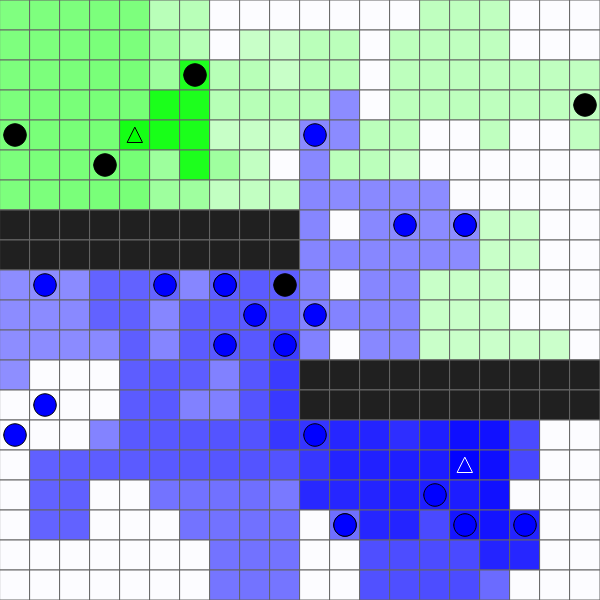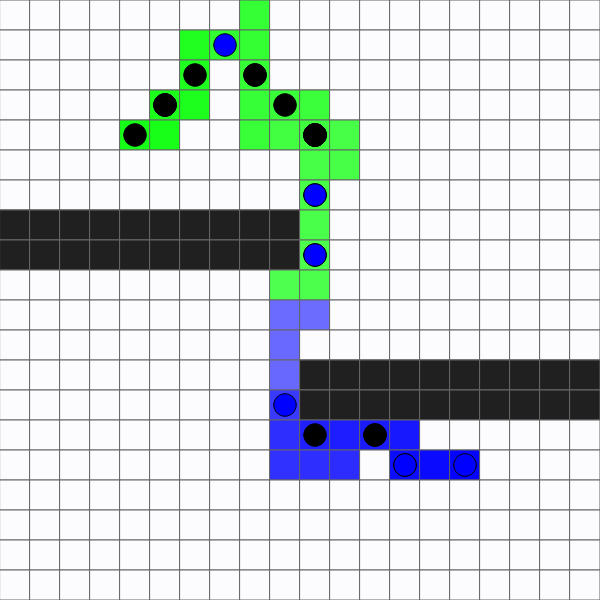# Ant Colony

example p5.js javascript creating-classes genuary

``````const columns = 20;
const rows = 20;

const nestC = 4;
const nestR = 4;

const foodC = 15;
const foodR = 15;

const maxAnts = 25;

const minPheromone = 1;
const maxPheromone = 100;
const evaporation = .99;
const dropoff = 0.99;
const trailStrength = 5; // 1 - 10+
const antFrameRate = 60; // 1 - 60

const ants = [];
const grid = [];
let cellWidth;
let cellHeight;

function setup() {
createCanvas(400, 400);

cellWidth = width / columns;
cellHeight = height / rows;

for (let c = 0; c < columns; c++) {
grid[c] = [];

for (let r = 0; r < rows; r++) {
grid[c][r] = new Cell(c, r);
}
}

grid[foodC][foodR].foodAmount = 10;

for (let i = 0; i < maxAnts; i++) {
ants.push(new Ant());
}
}

function draw() {
background(220);

// step and draw the grid
for (let c = 0; c < columns; c++) {
for (let r = 0; r < rows; r++) {
if (frameCount % floor(60 / antFrameRate) == 0) {
grid[c][r].step();
}
grid[c][r].draw();
}
}

// step and draw the ants
for (const ant of ants) {
if (frameCount % floor(60 / antFrameRate) == 0) {
ant.step();
}
ant.draw();
}

if(mouseIsPressed){
const mouseC = floor(mouseX / cellWidth);
const mouseR = floor(mouseY / cellHeight);
if(mouseC >= 0 && mouseC < columns && mouseR >=0 && mouseR < rows){
grid[mouseC][mouseR].obstacle = true;
}
}
}

class Ant {
constructor() {
this.c = nestC;
this.r = nestR;
this.goingHome = false;
}

step() {

const neighbors = [];
let totalChance = 0;
let maxNeighborFoodPheromone = 0;
let maxNeighborHomePheromone = 0;

// sniff the pheromones of neighbor cells
for (const cell of grid[this.c][this.r].getNeighbors()) {
const chance = pow(this.goingHome ?
cell.homePheromone : cell.foodPheromone,
trailStrength);
neighbors.push({
chance,
cell
});
totalChance += chance;

if (cell.homePheromone > maxNeighborHomePheromone) {
maxNeighborHomePheromone = cell.homePheromone;
}

if (cell.foodPheromone > maxNeighborFoodPheromone) {
maxNeighborFoodPheromone = cell.foodPheromone;
}
}

// release pheromones in the current cell
const cell = grid[this.c][this.r];

if (this.c == nestC && this.r == nestR) {
this.goingHome = false;
cell.homePheromone = maxPheromone;
} else {
cell.homePheromone = maxNeighborHomePheromone * dropoff;
}

if (cell.foodAmount > 0) {
this.goingHome = true;
cell.foodPheromone = maxPheromone;
} else {
cell.foodPheromone = maxNeighborFoodPheromone * dropoff;
}

// choose the next cell
const chance = random(totalChance);
let currentChance = 0;
for (const cell of neighbors) {
currentChance += cell.chance;
if (chance < currentChance) {
this.c = cell.cell.c;
this.r = cell.cell.r;
break;
}
}
}

draw() {
const x = this.c * cellWidth + cellWidth / 2;
const y = this.r * cellHeight + cellHeight / 2;

if (this.goingHome) {
fill(0, 0, 255);
} else {
fill(0);
}

stroke(0);
ellipse(x, y, cellWidth * .75, cellHeight * .75);
}
}

class Cell {
constructor(c, r) {
this.c = c;
this.r = r;
this.homePheromone = 0;
this.foodPheromone = 0;
this.foodAmount = 0;
this.obstacle = false;

this.x = this.c * cellWidth;
this.y = this.r * cellHeight;
}

getNeighbors() {
let neighbors = [];
if (this.c > 0) {
neighbors.push(grid[this.c - 1][this.r]);
}
if (this.c < columns - 1) {
neighbors.push(grid[this.c + 1][this.r]);
}

if (this.r > 0) {
neighbors.push(grid[this.c][this.r - 1]);
}
if (this.r < rows - 1) {
neighbors.push(grid[this.c][this.r + 1]);
}

neighbors = neighbors.filter(cell => !cell.obstacle);

return neighbors;
}

step() {
this.homePheromone *= evaporation;
this.homePheromone = constrain(this.homePheromone, minPheromone, maxPheromone);

this.foodPheromone *= evaporation;
this.foodPheromone = constrain(this.foodPheromone, minPheromone, maxPheromone);
}

draw() {
let cellColor;

if (this.homePheromone > this.foodPheromone) {
cellColor = lerpColor(color(255), color(0, 255, 0),
this.homePheromone / maxPheromone);
} else {
cellColor = lerpColor(color(255), color(0, 0, 255),
this.foodPheromone / maxPheromone);
}

stroke(100);
fill(cellColor);
rect(this.x, this.y, cellWidth, cellHeight);

// draw the nest
if(this.c == nestC && this.r == nestR){
stroke(0);
fill(0, 255, 0);
triangle(this.x + cellWidth * .5, this.y + cellHeight * .25,
this.x + cellWidth * .75, this.y + cellHeight * .75,
this.x + cellWidth * .25, this.y + cellHeight * .75);
}

// draw the food
if (this.foodAmount > 0) {
stroke(255);
fill(0, 0, 255);
triangle(this.x + cellWidth * .5, this.y + cellHeight * .25,
this.x + cellWidth * .75, this.y + cellHeight * .75,
this.x + cellWidth * .25, this.y + cellHeight * .75);
}

if(this.obstacle){
fill(32);
rect(this.x, this.y, cellWidth, cellHeight);
}
}

}
``````

This sketch implements a version of an ant colony optimization algorithm. Ants start out at their nest and leave behind pheromones as they travel around the grid. When any ant finds food, it uses those pheromones to travel back to the nest, and it leaves behind new pheromones so other ants can find the food.

I created this for the 30th day of #genuary which had a prompt of “Replicate a natural concept (e.g. gravity, flocking, path following).”

Ants start out travelling randomly, leaving behind pheromones based on when they saw the nest or the food: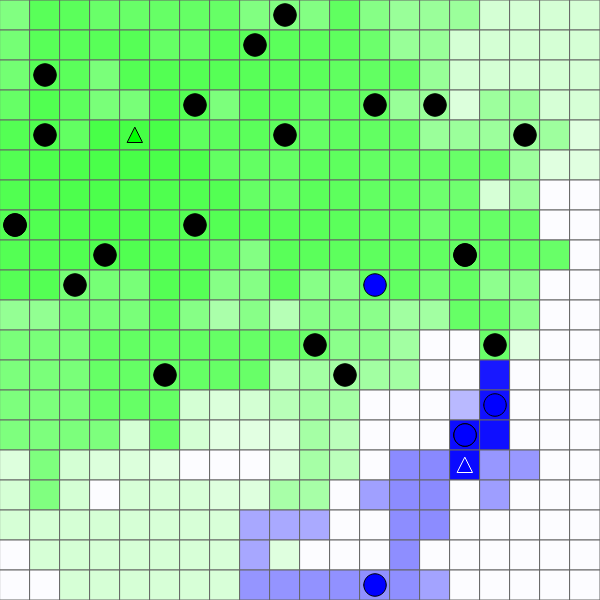Over time, ants use these pheromone trails to find a path between the nest and the food: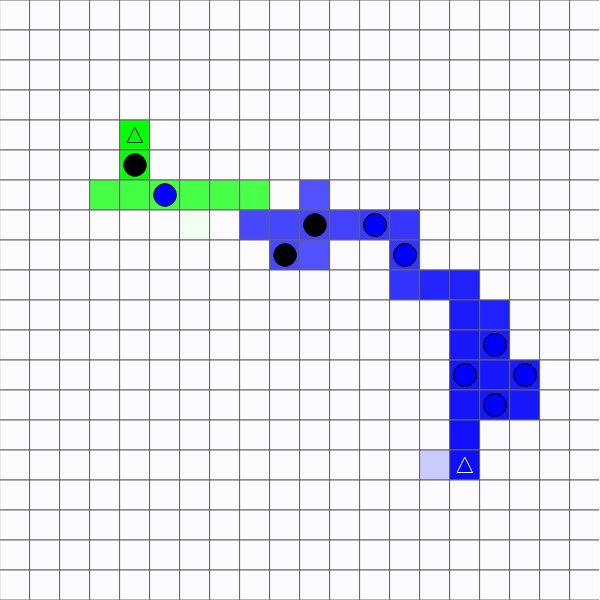If an obstacle is added, ants go back to travelling around randomly: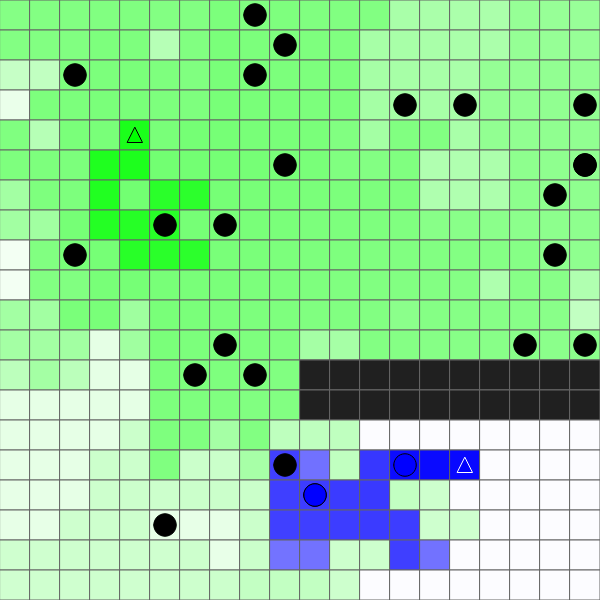Eventually they use the pheromones to find a new trail: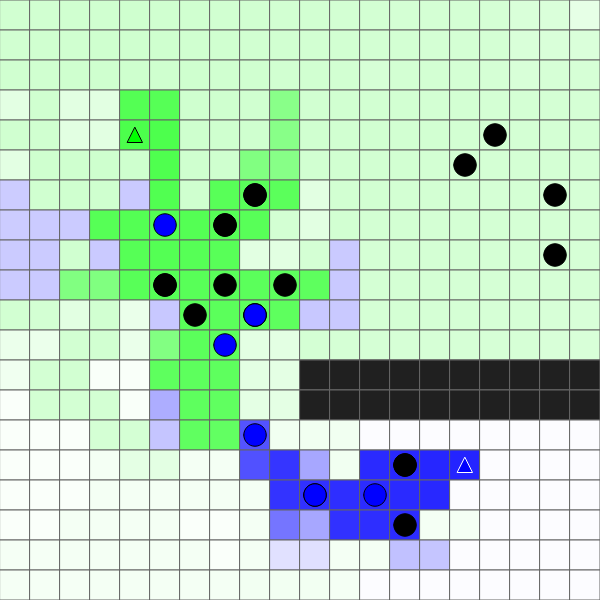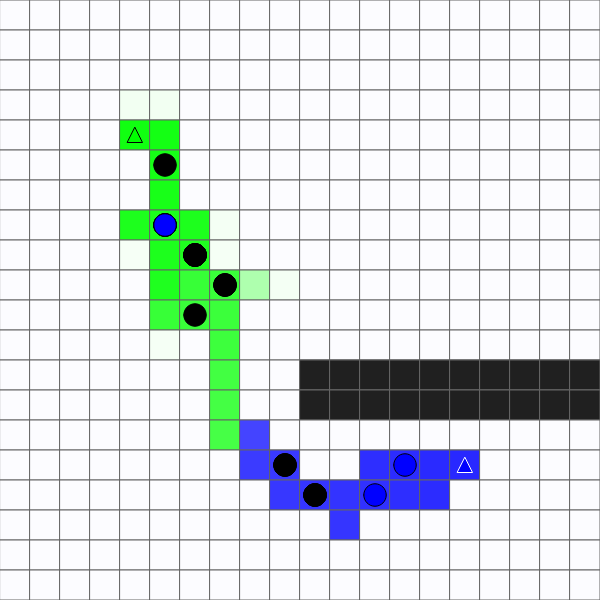Each individual ant follows a set of simple rules, but the colony can adapt to changes in the environment: